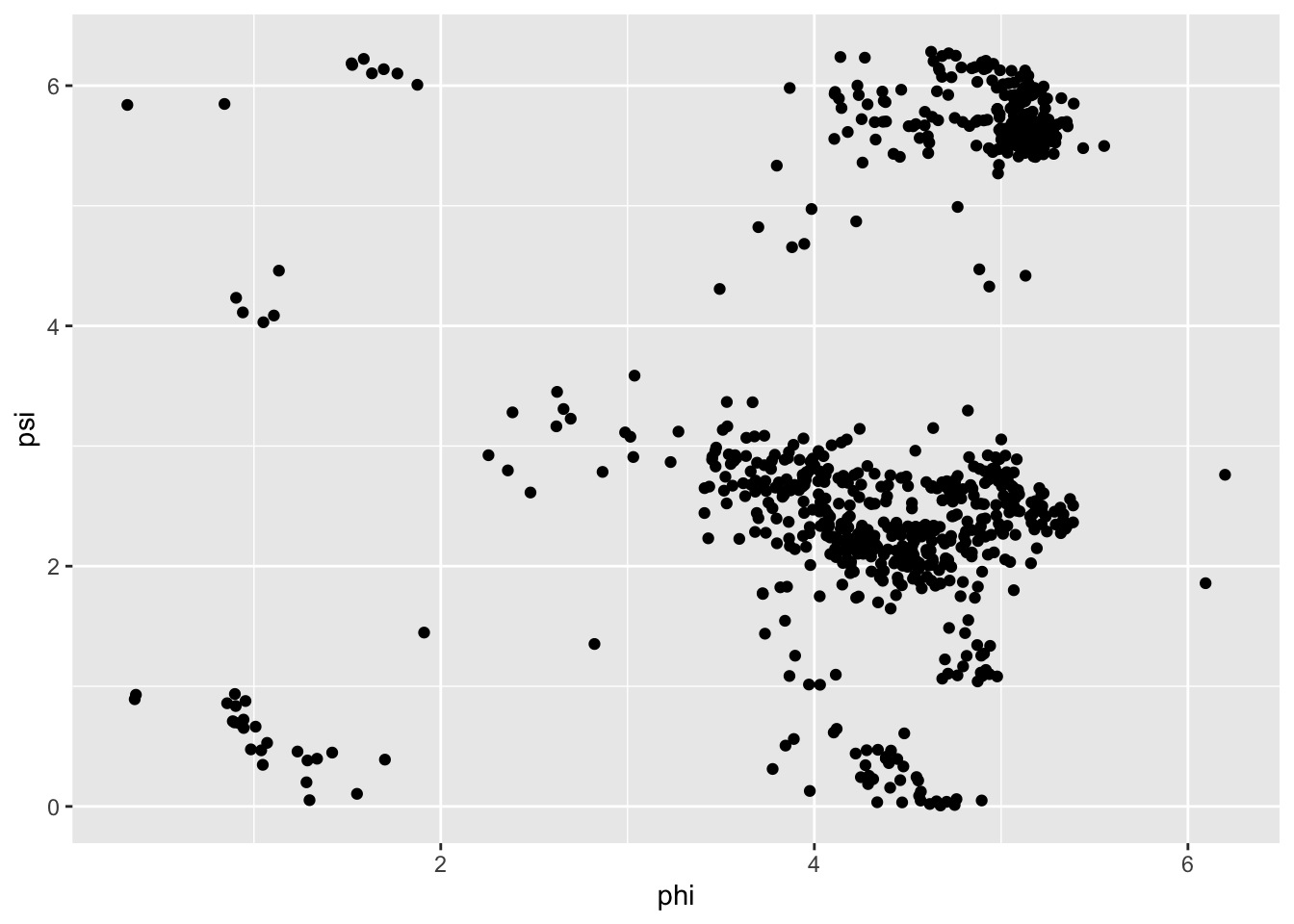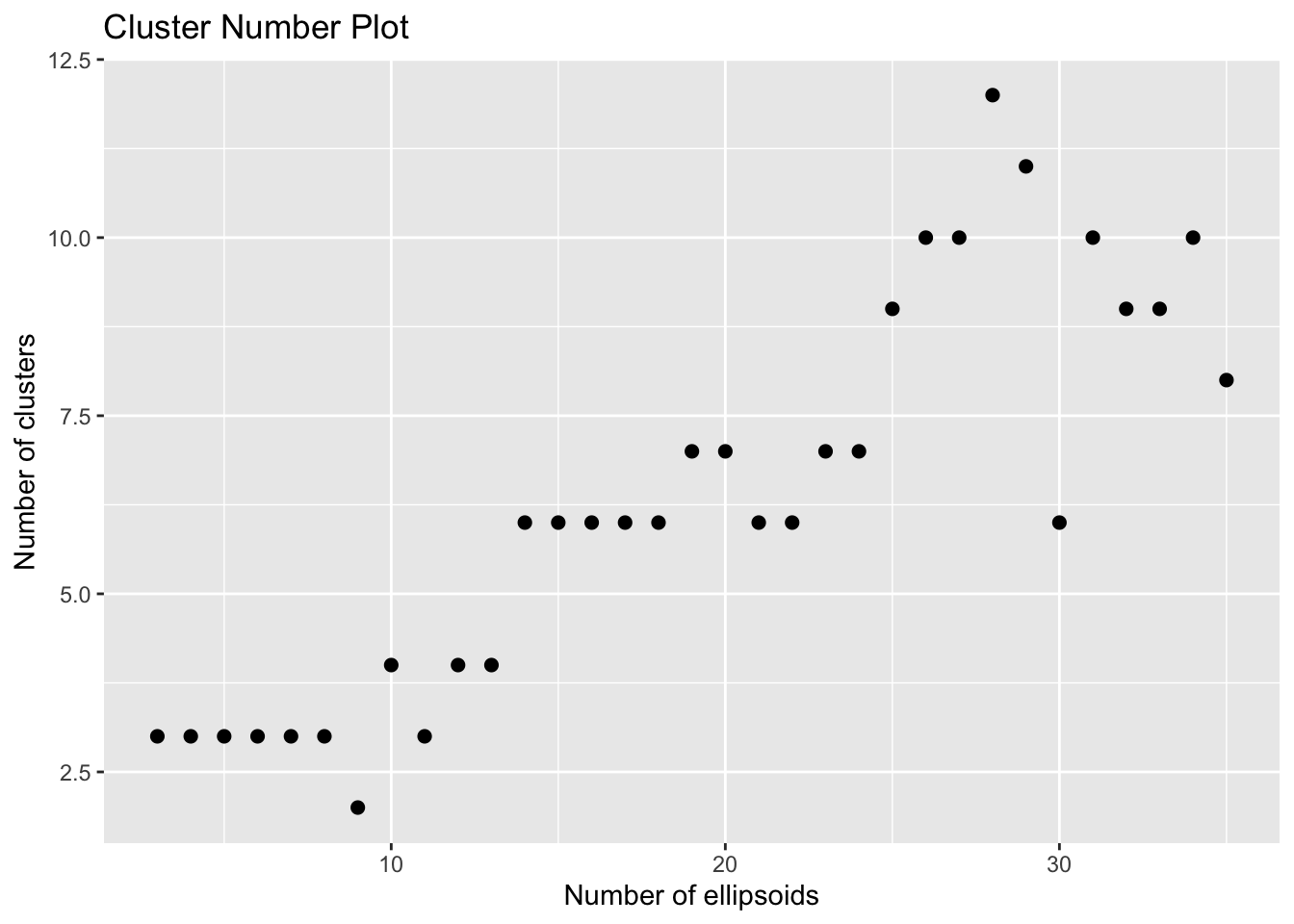ClusTorus is a package for clustering angular data, especially for protein structure data. ClusTorus provides various clustering algorithms designed with conformal prediction framework, which can deal with the outliers. The package suggests various methods for fitting the algorithms, and some of them will be introduced soon. The package also provides some simple tools for handling angluar data, such as angular subtraction, computing angular distance, etc. Now, check how to use ClusTorus briefly.

## Data Loading and Handling

ClusTorus provides a protein structure data of SARS-CoV-2, which provoked the recent pandemic situation with COVID-19. With this data, we will introduce how to load the data and how to use data handling tools of ClusTorus. Since SARS-CoV-2 data is originally extracted from protein data bank, it contains various information for describing protein structure. For our purpose, we will only use SARS_CoV_2$tbl, which only contains angluar information of the data. Moreover, for simplicity, we will only use backbone-chain angles, that is, $$\phi$$ and $$\psi$$ columns. library(ClusTorus) library(tidyverse) data <- SARS_CoV_2$tbl
data <- data.frame(data, id = rownames(data)) %>%
separate(id,into = c(',','position','type','rest'))
data <- data %>% select(-",")

data <- data[(datatype == "B"), ] data <- na.omit(data[, 1:2]) head(data) #> phi psi #> 27.B.ALA -52.38603 146.62861 #> 28.B.TYR -134.44609 152.58700 #> 29.B.THR -134.15780 175.49552 #> 30.B.ASN -89.74427 117.60396 #> 31.B.SER -71.24783 -15.14771 #> 32.B.PHE 51.81731 -117.41821 To use the functions of ClusTorus, we need to convert the data to be on radian scale, especially on $$[0, 2\pi)$$. data <- data / 180 * pi data <- on.torus(data) ggplot(data = as.data.frame(data), mapping = aes(x = phi, y = psi)) + geom_point()on.torus converts the radian scale data to be on $$[0, 2\pi)^d$$, where $$d$$ means the number of dimension. In this case, $$d = 2$$ and thus the data is converted to be on $$[0, 2\pi)^2$$. ## Clustering with Various Options Now, we are ready to implement clustering algorithms to the data. ClusTorus provides various options for clustering, but we will provide only several cases: “kde”, “mixture - axis aligned”, and “kmeans - general”. On the other hand, we need to choose hyperparameters: the number of modes or ellipsoids $$J$$ and the significance level $$\alpha$$. Before choosing the hyperparameter, we will implement the model fitting function icp.torus.score with various hyperparameter options, first. set.seed(2021) Jvec <- 3:35 l <- list() for (j in Jvec){ l[[j]] <- icp.torus.score(as.matrix(data), method = "all", mixturefitmethod = "axis-aligned", kmeansfitmethod = "general", param = list(J = j, concentration = 25), verbose = FALSE) } The list l contains the icp.torus objects, which consist of fitted parameters for generating clusters, by varying the hypterparameter $$J$$. That is, These objects are optimally fitted ingredients for generating clusters for given $$J$$. By specifying the significance level, we can get the clusters. But, how to generate optimal clusters? One may think that the hyperparameter which generates the cluster of the minimum volume/area will be the optimum for given significance level. For dealing with such volume/area based hyperparameter searching, ClusTorus provides icp.torus.eval function, which generates boolean matrices for indicating the inclusion of pre-specified evaluation points for given significance level(s). You need to know that the result also changes by varying $$\alpha$$. Thus, we need to carefully choose $$\alpha$$ for better performance under pre-specified hyperparameter-choosing criterion. But, for simplicity, we will assume that the significance level $$\alpha$$ is given with $$0.1$$. Then, we can simply get the results by using pre-obtained icp.torus objects as below: alpha <- 0.1 out <- data.frame() for (j in Jvec){ a<-icp.torus.eval(l[[j]], level = alpha, eval.point = grid.torus()) mu_kde <- sum(aChat_kde[,1])
mu_e <- sum(a$Chat_e[,1]) mu_kmeans <- sum(a$Chat_kmeans[,1])
out <- rbind(out, data.frame(J = j, mu_kde = mu_kde, mu_e = mu_e, mu_kmeans = mu_kmeans))
}

head(out)
#>   J mu_kde mu_e mu_kmeans
#> 1 3   1619 2412      2239
#> 2 4   1372 2376      2024
#> 3 5   1601 2245      1645
#> 4 6   1483 1866      2253
#> 5 7   1799 2130      1853
#> 6 8   1578 2150      1703

We count the number of points included in the generated clusters. In this 2-dimensional case, grid.torus() generates the 10,000 grid points in default. That is, icp.torus.eval generates the matrices for indicating whether each grid point is included in the clusters. By counting the included points, we can approximately estimate the volume of clusters. mu_kde shows the volume of cluster generated by option kde, mu_e by option mixture with axis-aligned, and mu_kmeans by option kmeans with general. Now, with “minimum volume” criterion, choose optimal $$J$$ for each method, and generate the clusters.

J_kde <- out$J[which.min(out$mu_kde)]
J_e <- out$J[which.min(out$mu_e)]
J_kmeans <- out$J[which.min(out$mu_kmeans)]

icp.torus.kde <- l[[J_kde]]
icp.torus.mix <- l[[J_e]]
icp.torus.kmeans <- l[[J_kmeans]]

c_mix <- cluster.assign.torus(data, icp.torus.mix, level = alpha)
c_kmeans <- cluster.assign.torus(data, icp.torus.kmeans, level = alpha)

If there are some difficulties for evaluating such volume or for implementing your hyperparameter-choosing criterion, ClusTorus alternatively suggests the function cluster.assign.number, which generates the table of the number of modes/ellipsoids $$J$$ and the generated cluster number for given $$J$$. Moreover, for visual check, it also provides the visualization of the table. You may guess the true cluster number as the most frequent generated cluster number. For computational efficiency and the purpose of this function, it only provides method options of “kmeansfitmethod” of icp.torus.score. However, since this suggestion is not sophisticatedly(that is, mathematically) designed, you need to be careful to use this method. cluster.assign.number can be used as below:

cluster.num <- cluster.assign.number(data, Jmin = 3, Jmax = 35, method = "homogeneous-circular")
cluster.num$plotcluster.num$cluster.number %>% group_by(Number) %>% count()
#> # A tibble: 10 x 2
#> # Groups:   Number 
#>    Number     n
#>     <dbl> <int>
#>  1      2     1
#>  2      3     7
#>  3      4     3
#>  4      6     8
#>  5      7     4
#>  6      8     1
#>  7      9     3
#>  8     10     4
#>  9     11     1
#> 10     12     1

## Generating Clusters and Visualization of Clustering Results

With cluster.assign.torus, we can generate the cluster for option mixture and kmeans, and for each data point, the label of cluster is assigned. Moreover, for the cases of mixture and kmeans, we can check how the cluster is generated directly as below:

c_mix$mixture$plot
#> []